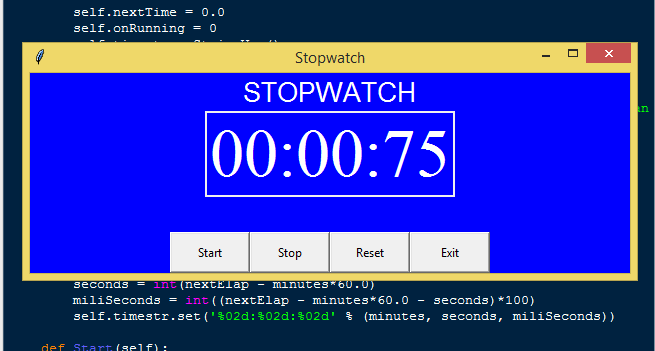# Introduction

In this tutorial we will create a How to Create Simple Stopwatch Program with Tkinter in Python. This tutorial purpose is to help you create simple program where you can track your physical record. This will cover all the basic parts for creating the stopwatch. I will provide a sample program to show the actual coding of this tutorial.

This tutorial will make it easy for you to understand just follow my instruction I provided and you can do it without a problem. This program is useful if you want to track your physical record, you can also set your speed using this stopwatch. I will try my best to give you the easiest way of creating this program Stopwatch. So let's do the coding.

## Importing Modules

After setting up the installation and the database, run the IDLE and click file and then new file. After that a new window will appear containing a black file this will be the text editor for the python. Then copy code that I provided below and paste it inside the IDLE text editor.
`from tkinter import *import tkinter.messagebox as tkMessageBoximport time`

## Creating Main Function

This is the main function of the application is. This code will create the stopwatch with a complete functionality. To do this just copy and write these block of codes inside the IDLE text editor.
`class StopWatch(Frame):       def __init__(self, parent=None, **kw):                Frame.__init__(self, parent, kw)        self.startTime = 0.0                self.nextTime = 0.0        self.onRunning = 0        self.timestr = StringVar()                       self.MakeWidget()           def MakeWidget(self):                                 timeText = Label(self, textvariable=self.timestr, font=("times new roman", 50), fg="white", bg="blue")        self.SetTime(self.nextTime)        timeText.pack(fill=X, expand=NO, pady=2, padx=2)                           def Updater(self):         self.nextTime = time.time() - self.startTime        self.SetTime(self.nextTime)        self.timer = self.after(50, self.Updater)     def SetTime(self, nextElap):        minutes = int(nextElap/60)        seconds = int(nextElap - minutes*60.0)        miliSeconds = int((nextElap - minutes*60.0 - seconds)*100)                        self.timestr.set('%02d:%02d:%02d' % (minutes, seconds, miliSeconds))     def Start(self):                                                             if not self.onRunning:                        self.startTime = time.time() - self.nextTime            self.Updater()            self.onRunning = 1             def Stop(self):                                            if self.onRunning:            self.after_cancel(self.timer)                        self.nextTime = time.time() - self.startTime                self.SetTime(self.nextTime)            self.onRunning = 0     def Exit(self):        result = tkMessageBox.askquestion('System Information', 'Are you sure you want to exit?', icon='warning')        if result == 'yes':            root.destroy()            exit()     def Reset(self):                                          self.startTime = time.time()                 self.nextTime = 0.0            self.SetTime(self.nextTime) def Main():    global root     root = Tk()    root.title("Stopwatch")    width = 600    height = 200    screen_width = root.winfo_screenwidth()    screen_height = root.winfo_screenheight()    x = (screen_width / 2) - (width / 2)    y = (screen_height / 2) - (height / 2)    root.geometry("%dx%d+%d+%d" % (width, height, x, y))    Top = Frame(root, width=600)    Top.pack(side=TOP)    stopWatch = StopWatch(root)    stopWatch.pack(side=TOP)    Bottom = Frame(root, width=600)    Bottom.pack(side=BOTTOM)    Start =  Button(Bottom, text='Start', command=stopWatch.Start, width=10, height=2)    Start.pack(side=LEFT)    Stop = Button(Bottom, text='Stop', command=stopWatch.Stop, width=10, height=2)    Stop.pack(side=LEFT)    Reset = Button(Bottom, text='Reset', command=stopWatch.Reset, width=10, height=2)    Reset.pack(side=LEFT)    Exit = Button(Bottom, text='Exit', command=stopWatch.Exit, width=10, height=2)    Exit.pack(side=LEFT)    Title = Label(Top, text="STOPWATCH", font=("arial", 20), fg="white", bg="blue")    Title.pack(fill=X)    root.config(bg="blue")    root.mainloop() `

In the given code we created multiple methods called Start, Stop, SetTime and Updater. In the SetTime we set the timer in minutes, seconds, and milliseconds format with a corresponding formula for getting the value. We then create Start function, this will trigger the Updater to start the timer. The Updater function will continuously run the timer after you start it, by the difference of time.time() and StartTime value. Lastly, the Stop function will immediately top the timer when called.

## Initializing the Application

After finishing the function save the application as index.py. This function will run the code and check if the main is initialize properly. To do that copy and write these block code after the main function inside the IDLE text editor.

`if __name__ == '__main__':    Main()`

## Output: